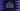# C++ read string with spaces from console### Introduction :

We mostly use cin method to read user inputs in C++. cin() works great if you are reading a character, float or integer. But, if you read a string using cin() it will fail to read the complete string if it has a space in between the words.

For example :

``````#include <iostream>
using namespace std;

int main()
{
char user_input = {};

cout<<"Enter a string : "<<endl;
cin >> user_input;

cout<<"You have entered : "<<user_input<<endl;

return 0;
}``````

If you run this program, you will get outputs as like below :

``````Enter a string :
hello
You have entered : hello

Enter a string :
hello world
You have entered : hello``````

In the second example, we entered hello world, but it reads only the first word hello. That is the problem with cin.

## Use getline() to read string with spaces :

getline() is defined in std::istream class. It reads a string and stores it in a variable as a c-string. This method is defined as below :

``````getline (char* s, streamsize n )
getline (char* s, streamsize n, char delim )``````
• s is the pointer to an array of characters. getline reads the string and stores the content as a c-string in s.
• n is the maximum number of characters to write to the string s.
• delim is the delimiting character to add to the string. If we use the first version, it will add one newline automatically to the final string.

### Example to use getline() :

The below example shows how to use getline() :

``````#include <iostream>
using namespace std;

int main()
{
char user_input = {};

cout<<"Enter a string : "<<endl;
cin.getline(user_input,30);

cout<<"You have entered : "<<user_input<<endl;

return 0;
}``````

This time, it will read a string irrespective of the blank space in between words.

``````Enter a string :
Hello World
You have entered : Hello World``````

If the streamsize is less than the size of the string, it will not read the complete string.

### Example of streamsize less than the string size :

Let’s consider the below example :

``````#include <iostream>
using namespace std;

int main()
{
char user_input = {};

cout<<"Enter a string : "<<endl;
cin.getline(user_input,5);

cout<<"You have entered : "<<user_input<<endl;

return 0;
}``````

This is similar to the above example. The only difference is that I am using 5 as the streamsize.

Here, it will assign only four characters from the user input and add one newline i.e. total five characters to the character array user_input.

``````Enter a string :
hello world
You have entered : hell``````